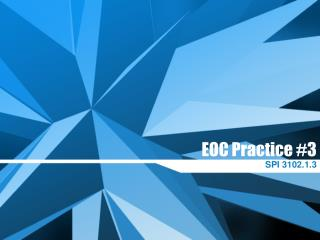Download PresentationEOC Practice #3

# EOC Practice #3 - PowerPoint PPT Presentation

EOC Practice #3. SPI 3102.1.3. EOC Practice #3. Apply properties to evaluate expressions, simplify expressions, and justify solutions to problems. Simplify: 3(x – 2y) + 7(x + 3y) – 3y A. 4x – 30y B. 10x + 12y C. – 6x + 12y D. 7x – 10y.I am the owner, or an agent authorized to act on behalf of the owner, of the copyrighted work described.
Download Presentation## EOC Practice #3

An Image/Link below is provided (as is) to download presentation

Download Policy: Content on the Website is provided to you AS IS for your information and personal use and may not be sold / licensed / shared on other websites without getting consent from its author.While downloading, if for some reason you are not able to download a presentation, the publisher may have deleted the file from their server.

- - - - - - - - - - - - - - - - - - - - - - - - - - E N D - - - - - - - - - - - - - - - - - - - - - - - - - -
Presentation Transcript
1. EOC Practice #3 SPI 3102.1.3

2. EOC Practice #3 • Apply properties to evaluate expressions, simplify expressions, and justify solutions to problems.

3. Simplify: • 3(x – 2y) + 7(x + 3y) – 3y • A. 4x – 30y • B. 10x + 12y • C. –6x + 12y • D. 7x – 10y

4. Simplify: • –4(2x – 3y) + 4x – 2(x + 6) • A. –6x • B. –6x + 24y • C. 12y – 12 • D. –6x + 12y – 12

5. If x = –2, y = 5, and z = 3, • evaluate the following expression. • x4– 5y + 2(x – z)2 • A. 50 • B. –41 • C. 41 • D. 63

6. Simplify: • 5x6(2x4– x3 + 7x2– 4x) • A. 10x10– 5x9 + 35x8– 20x7 • B. 10x24– 5x18 + 35x12– 20x6 • C. 7x24– 4x18 + 12x12 + x6 • D. 20x34

7. 5. If x = –4 and y = 8, evaluate the following expression. A. 1544 B. –1500 C. –200 D. 1540

8. Identify the property used to • simplify the following expression. • 3(x – 7) = 3x – 21 • A. Associative Property of Addition • B. Commutative Property of Addition • C. Distributive Property • D. Identity Property of Addition

9. What is the value of the • expression when x = 6 and • y = –4? • 8xy2 – 5x2 • A. –948 • B. 588 • C. 324 • D. 35,964

10. David wants to buy a new bicycle • that cost \$295 before a 40% • discount. He finds the cost after • the discount, in dollars, by • evaluating 295 – 295(0.40). His • brother Michael finds the same cost • by evaluating 295(1 – 0.40). What • property can be used to justify that • these two expressions represent • the same cost after the discount?

11. A. associative property B. commutative property C. distributive property D. subtraction property of equality

13. Simplify: • 3(x – 2y) + 7(x + 3y) – 3y • A. 4x – 30y • B. 10x + 12y • C. –6x + 12y • D. 7x – 10y

14. Simplify: • –4(2x – 3y) + 4x – 2(x + 6) • A. –6x • B. –6x + 24y • C. 12y – 12 • D. –6x + 12y – 12

15. If x = –2, y = 5, and z = 3, • evaluate the following expression. • x4– 5y + 2(x – z)2 • A. 50 • B. –41 • C. 41 • D. 63

16. Simplify: • 5x6(2x4– x3 + 7x2– 4x) • A. 10x10– 5x9 + 35x8– 20x7 • B. 10x24– 5x18 + 35x12– 20x6 • C. 7x24– 4x18 + 12x12 + x6 • D. 20x34

17. 5. If x = –4 and y = 8, evaluate the following expression. A. 1544 B. –1500 C. –200 D. 1540

18. Identify the property used to • simplify the following expression. • 3(x – 7) = 3x – 21 • A. Associative Property of Addition • B. Commutative Property of Addition • C. Distributive Property • D. Identity Property of Addition

19. What is the value of the • expression when x = 6 and • y = –4? • 8xy2 – 5x2 • A. –948 • B. 588 • C. 324 • D. 35,964

20. David wants to buy a new bicycle • that cost \$295 before a 40% • discount. He finds the cost after • the discount, in dollars, by • evaluating 295 – 295(0.40). His • brother Michael finds the same cost • by evaluating 295(1 – 0.40). What • property can be used to justify that • these two expressions represent • the same cost after the discount?

21. A. associative property B. commutative property C. distributive property D. subtraction property of equality